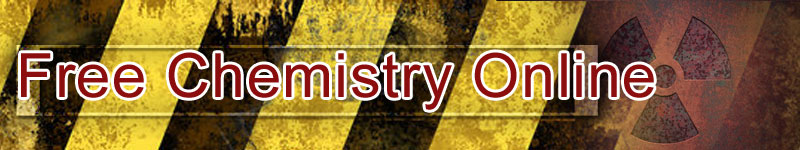## Atomic Symbol

The symbol of an atom can be written to denote its number (number of protons) and its mass number (sum of number of protons and neutrons) as shown: AZX where X represents the atom, A represents the mass number and Z represents the atomic number.

Note: 1. Atoms of all elements except hydrogen contain three particles. Namely: protons, electrons, and neutrons. In the case of hydrogen, only a proton and an electron are contained. Neutron is absent.

2. An atom has equal number of protons and electrons – it is therefore neutral. For example, aluminium atom, 2713Al contains 13 protons (atomic number) and 13 electrons. Since the mass (27) is equal to number of protons + number of neutrons, then number of neutrons = 27 - 13 = 14.

3. If an atom loses electrons, its number of protons increases more than the electrons by the number of electrons lost, hence, it becomes a positively charged particle called cation – its number of charge equal the number of electrons lost.

For example, 2713Al3+ contains 13 protons and 3 electrons less than the protons (i.e. 10 electrons). Number of neutrons remain as 14.

4. If an atom gains electrons, its number of electrons increases more than the protons by the number of electrons gained, hence it becomes a negatively charged particle called anion, with it number of charge equaling the number of electrons gained. For example, oxygen ion, 168O2- contains 8 protons and 2 electrons more than the protons (i.e. 10 electrons). Number of neutrons = 16 - 8 = 8.

5. Either loss or gain of electrons, the electronic configuration changes to reflect the loss or gain.

 Like This Post? Please Share!!

Copyright , All Rights Reserved Free Chemistry Online | About Us | Usage of Content | Total Disclosures | Privacy Policy